# Colleges with the highest SAT scores in Tennessee

Top 10 colleges in Tennessee with the highest SAT scores
Looking for the colleges with the highest SAT scores in Tennessee? Well you're in luck! We've compiled a national college database and have created a list of the top 10 universities with the highest SAT scores in Tennessee. These are the schools whose applicants had the highest average SAT scores in Tennessee. And, since these tests are meant to determine academic prowess, they are arguably the schools with the most academically proficient students. You could even say these are the best colleges in Tennessee. We also include each college's ACT scores and acceptance rate so that you can see where you would have the easiest or hardest time getting in. Read on to find out more.

## Vanderbilt University SAT scores

The average SAT score for Vanderbilt University is 1505.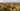The average SAT score of 1505 breaks down into:

• SAT math: 775

The average ACT score for Vanderbilt University is 34 and their acceptance rate is 9.6%.

## Rhodes College SAT scores

The average SAT score for Rhodes College is 1330.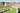The average SAT score of 1330 breaks down into:

• SAT math: 660

The average ACT score for Rhodes College is 29 and their acceptance rate is 44.6%.

## Sewanee The University of the South SAT scores

The average SAT score for Sewanee The University of the South is 1286.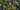The average SAT score of 1286 breaks down into:

• SAT math: 640

The average ACT score for Sewanee The University of the South is 28 and their acceptance rate is 65.2%.

## The University of Tennessee Knoxville SAT scores

The average SAT score for The University of Tennessee Knoxville is 1240.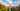The average SAT score of 1240 breaks down into:

• SAT math: 620

The average ACT score for The University of Tennessee Knoxville is 27 and their acceptance rate is 78.8%.

## Belmont University SAT scores

The average SAT score for Belmont University is 1225.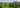The average SAT score of 1225 breaks down into:

• SAT math: 600

The average ACT score for Belmont University is 27 and their acceptance rate is 83.8%.

## Milligan College SAT scores

The average SAT score for Milligan College is 1198.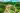The average SAT score of 1198 breaks down into:

• SAT math: 600

The average ACT score for Milligan College is 25 and their acceptance rate is 83.4%.

## Christian Brothers University SAT scores

The average SAT score for Christian Brothers University is 1180.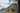The average SAT score of 1180 breaks down into:

• SAT math: 590

The average ACT score for Christian Brothers University is 24 and their acceptance rate is 56%.

## Union University SAT scores

The average SAT score for Union University is 1178.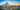The average SAT score of 1178 breaks down into:

• SAT math: 580

The average ACT score for Union University is 26 and their acceptance rate is 60%.

## Freed-Hardeman University SAT scores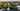The average SAT score of 1140 breaks down into:

• SAT math: 560

The average ACT score for Freed-Hardeman University is 24 and their acceptance rate is 96.3%.

## Middle Tennessee State University SAT scores

The average SAT score for Middle Tennessee State University is 1140.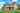The average SAT score of 1140 breaks down into:

• SAT math: 560